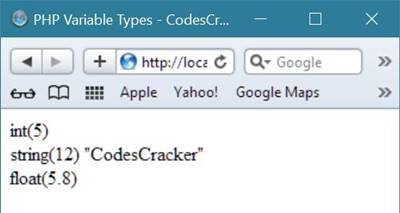# PHP Variable Types

In PHP language, when you initialize some value to a variable, for example, if you initialize an integer value say 5 to a variable \$num, then the \$num variable can be called as of integer type variable, and in next line when you initialize a string value say "CodesCracker" to the same variable, that is, \$num, then this time the variable called \$num now become of string type variable.

In other words, you can say that in PHP, the variable type is totally depends on the value contained by the variable, that is, if a variable contains integer value, then that variable is of integer type, and if contains a string value, then that variable became of string type, same in case of double or float, and in other.

## PHP Variable Type Example

Let's take an example to understand how this works or the working of PHP variable type.

```<html>
<title>PHP Variable Types - CodesCracker</title>
<body>
<?php
# PHP Variable Types Example - CodesCracker
\$num = 5;
echo var_dump(\$num);
echo "<br/>";
\$num = "CodesCracker";
echo var_dump(\$num);
echo "<br/>";
\$num = 5.8;
echo var_dump(\$num);
echo "<br/>";
?>
</body>
</html>```

Here is the sample output produced by the above PHP variable type example code:Tools
Calculator